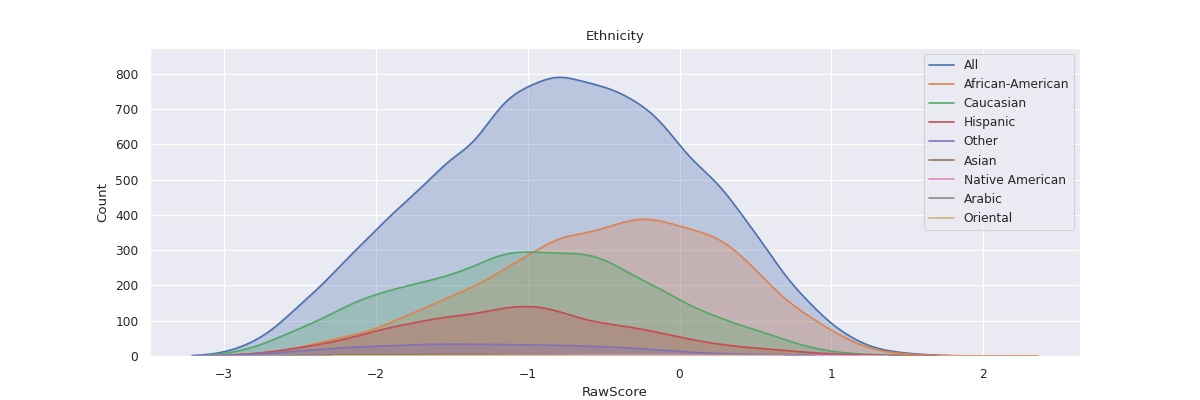# fairlens.plot.attr_distr_plot#

attr_distr_plot(df, target_attr, attr, distr_type=None, attr_distr_type=None, max_quantiles=8, separate=False, show_hist=None, show_curve=None, shade=True, normalize=False, cmap=None, ax=None)[source]#

Plot the distribution of the target attribute with respect to all the unique values in the column attr.

Parameters
• df (pd.DataFrame) – The input dataframe.

• target_attr (str) – The target attribute.

• attr (str) – The attribute whose values’ distributions are to be plotted.

• distr_type (Optional[str], optional) – The type of distribution of the target attribute. Can take values from [“categorical”, “continuous”, “binary”, “datetime”]. If None, the type of distribution is inferred based on the data in the column. Defaults to None.

• attr_distr_type (Optional[str], optional) – The type of distribution of attr. Can be “categorical”, “continuous” or “datetime”. If None the type of distribution is inferred based on the data in the column. Defaults to None.

• max_quantiles (int, optional) – The maximum amount of quantiles to use for continuous data. Defaults to 8.

• separate (bool, optional) – Separate into multiple plots (subplot). Defaults to False.

• show_hist (Optional[bool], optional) – Shows the histogram if True. Defaults to True if the data is categorical or binary.

• show_curve (Optional[bool], optional) – Shows a KDE if True. Defaults to True if the data is continuous or a date.

• shade (bool, optional) – Shades the curve if True. Defaults to True.

• normalize (bool, optional) – Normalizes the counts so the sum of the bar heights is 1. Defaults to False.

• cmap (Optional[Sequence[Tuple[float, float, float]]], optional) – A sequence of RGB tuples used to colour the histograms. If None seaborn’s default pallete will be used. Defaults to None.

• ax (Optional[matplotlib.axes.Axes], optional) – An axis to plot the figure on. Defaults to plt.gca(). Defaults to None.

Returns

The matplotlib axes containing the plot if separate is False, otherwise None.

Return type

Optional[matplotlib.axes.Axes]

Examples

```>>> df = pd.read_csv("datasets/compas.csv")
>>> attr_distr_plot(df, "RawScore", "Ethnicity")
>>> plt.show()
```